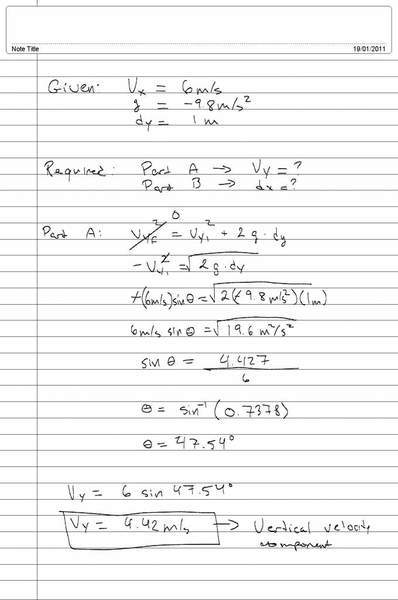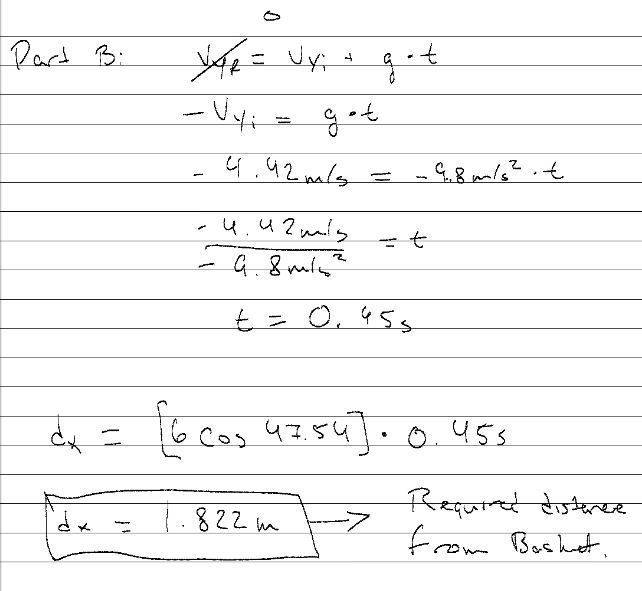chris_0101
1. Homework Statement [/b

A basketball player is approaching the basket at a velocity of 6m/s when he jumps into the air to perform a slam dunk. Calculate the vertical velocity component required to lift the player 1m above the ground. Determine the distance from the basket needed for the start of the jump in order for the player to reach the maximum height at the position of the basket

Attached to this thread is my solution, however the wording of the question is puzzling me and I do not know if the 1m that is mentioned is a point during his jump or the maximum height of his jump. My solution is based off of assuming that the 1m mentioned is the maximum height.

Basically I am asking if my solution is right or wrong and if anyone can point me into the right direction,

Thanks

## Homework Equations

All relevant equations are listed in the attached files

## The Attempt at a Solution

See the attached files below:Thanks for the help